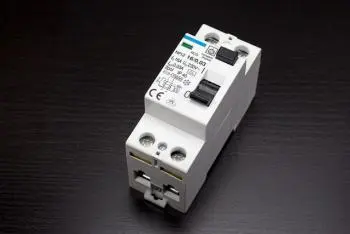# Power Factor: Definition, Formula, Calculation and CorrectionIn electricity, power factor is a measure of the efficiency in transferring electrical energy from a power source to a load. It is defined as the ratio between active power (measured in watts) and apparent power (measured in volt-amperes).

Active power is the amount of energy that is actually consumed to do useful work, such as turning on a light bulb or running a machine. Apparent power, on the other hand, is the total amount of power that is supplied to the load, including the energy that is lost in the process of energy transfer due to electrical resistance, which is known as reactive power.

## Formula to Calculate the Power Factor?

The power factor (PF) is calculated by dividing the active power (P) by the apparent power (S) of an electrical circuit. Mathematically, it is expressed as:

FP = P/S

where:

• P is the active power in watts (W)

• S is the apparent power in volt-amperes (VA)

The result of this operation must be a value between 0 and 1.

A power factor close to 1 indicates that energy is being used efficiently, since most of the supplied energy is converted into useful work. On the other hand, a low power factor indicates that there is a significant amount of energy that is lost in the process of energy transfer.

## Power Factor Correction

There are several ways to correct the power factor and improve the energy efficiency of an electrical circuit. Here are some of the more common options:

1. Capacitor Installation: Capacitors are electrical devices that are used to store electrical energy in the form of an electric field. By adding capacitors to the circuit, the amount of reactive power consumed can be reduced, thereby increasing the power factor.

2. Use of transformers with high-quality magnetic cores: These transformers can help reduce the amount of reactive power consumed by electrical loads. These transformers are designed to minimize eddy currents and power losses, thus improving energy efficiency.

3. Switch to High Efficiency Motors: Electric motors are one of the most common loads that generate reactive power. By switching to high-efficiency motors, the amount of reactive power consumed can be reduced.

4. Electrical Load Reduction: In some cases, the electrical load in a circuit may be higher than necessary. By reducing the electrical load, the reactive power consumed is reduced. For example, electrical equipment that is not in use can be turned off or motors can be slowed down when they are not needed at full capacity.

5. Hiring power factor correction services: In some cases, it is possible to hire specialized services.

## How Does It Relate to the Electric Bill?

Power companies often charge a higher rate to consumers who have a low power factor. This is because low power factor consumers require more energy to do the same work as high power factor consumers.

In many countries, power companies charge a penalty to consumers who have a power factor below a set minimum value. This penalty is known as a reactive capacity charge, which is an additional fee that is applied to the electric bill to compensate for the energy that is lost due to low power factor. The lower the power factor, the greater the amount of power that is lost and therefore the higher the charge for reactive capacity.

## What Does the Power Factor Depend On?

The power factor can be influenced by the topology of the electrical circuit, the nature of the loads connected to the circuit, and the quality of the power supply.

Inductive loads, such as motors, transformers, and coils, are highly reactive in nature and can generate a significant amount of reactive power. Therefore, electrical circuits that power inductive loads often have a low power factor.

On the other hand, resistive loads, such as incandescent light bulbs and electric heaters, are resistive in nature and do not generate reactive power, so the electrical circuits that power these loads have a high power factor.

In general, good electrical circuit design, proper selection of electrical components, and use of power factor correction techniques can improve power factor and therefore increase efficiency in electrical energy transfer and reduce electricity costs.

## Consequences of a Low Power Factor

A low power factor can have several consequences, including:

• Increased energy losses: a significant proportion of the supplied energy is lost in the form of reactive power. These losses translate into a greater amount of energy consumed without generating useful work, resulting in lower energy efficiency.
• Higher Current Demand: To supply the same amount of active power to a load, a low power factor load will require a higher current compared to a high power factor load. This can result in overloaded cables and equipment, which can lead to increased wear and tear and safety issues.
• Additional costs on the electric bill: In many cases, electric companies apply additional charges to consumers with a low power factor. These charges are usually in the form of penalties or additional fees for the reactive energy consumed.
• Inefficiency in power distribution: the presence of reactive power can overload transformers and electrical conductors. This can result in a lower quality of electricity supply and increase the costs of maintaining and upgrading the electrical infrastructure.
• Limitations in the capacity of the electrical network: A low value can affect the ability of the electrical network to supply energy to other consumers, reduce the capacity available to other users and require additional investments in the electrical network.
Author:

Published: May 10, 2023
Last review: May 11, 2023